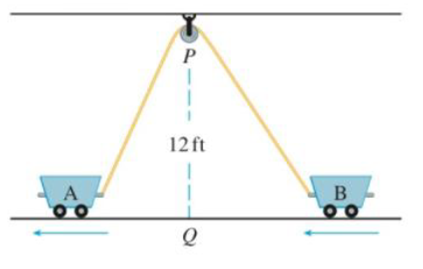Chapter 3.9, Problem 42E

Chapter
Section
Textbook Problem

Two carts, A and B, are connected by a rope 39 ft long that passes over a pulley P (see the figure). The point Q is on the floor 12 ft directly beneath P and between the carts. Cart A is being pulled away from Q at a speed of 2 ft/s. How fast is cart B moving toward Q at the instant when cart A is 5 ft from Q?To determine

To find: The rate at which the cart B is moving toward the point Q when cart A is 5 ft from Q.

Explanation

Given:

The two carts connected by a rope 39 ft long and pass over the pulley P .

The point Q is on the floor 12 ft directly below the point P and between the carts.

The rate of moving of the cart A away from the point Q is 2 ft/s.

Formula used:

(1) Chain rule:

(2) Pythagorean Theorem.

Calculation:

The two carts A and B connected by the 39 ft long rope and pass over the pulley P.

Let us assume that x be the distance between the cart A and the point Q and y be the distance between the cart B and the point Q as shown the figure 1 given below.

Let consider Q as the origin point.

Since the distance x and y changes with the time t.

Therefore, x and y are the function of the time t.

Since dxdt=2ft/s.

Obtain dydt when x=5ft from Q.

From the above figure 1 the total length is.

AB=AP+PB .

Then by using the Pythagorean Theorem in the triangle AQP and BQP

AB=x2+122+y2+122 .

Since the total length of the rope AB= 39 ft, therefore

x2+122+y2+122=39

Differentiate the total length of the rope with respect to the time t.

ddt[x2+122+y2+122]=ddtddx[x2+122]dxdt+ddy[y2+122]dydt=0[Qdydx=dydududx][12(x2+122)121(2x)]dxdt+[12(y2+122)121(2y)]dydt=0[xx2+122]dxdt+[yy2+122]dydt=0

On further simplification the rate of change speed of the cart Q is as follows

Still sussing out bartleby?

Check out a sample textbook solution.

See a sample solution

The Solution to Your Study Problems

Bartleby provides explanations to thousands of textbook problems written by our experts, many with advanced degrees!

Get Started

Find more solutions based on key concepts